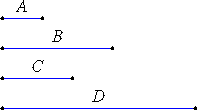# Definition 11

Antecedents are said to correspond to antecedents, and consequents to consequents.

# Definition 12

Alternate ratio means taking the antecedent in relation to the antecedent and the consequent in relation to the consequent.

# Definition 13

Inverse ratio means taking the consequent as antecedent in relation to the antecedent as consequent.

## GuideThe figure illustrates the proportion A : B = C : D. Thus, A and C are corresponding terms since they’re the antecedents. Also, B and D are corresponding terms since they’re the consequents. Note that for alternate ratios to exist, all four magnitudes must be of the same kind. The alternate ratios in this proportion are A : C and B : D. Euclid proves these are the same ratio in proposition V.16. Thereafter, given one proportion A : B = C : D, he concludes alternately the alternate proportion A : C = B : D.

The ratio inverse to A : B is B : A. It is evident from the definition V.Def.5 that A : B = C : D and B : A = D : C reduce to the same conditions on A, B, C, and D. Therefore, if two ratios are the same, then their two inverse ratios are also the same. For some reason, this statement is misplaced as the corollary after proposition V.7.

Several of the propositions are stated using the antecedent terms but they apply as well for the consequent terms by inversion. For example, proposition V.24 says that if u : v = w : x and y : v = z : x, then (u + y):v = (w + z):x. But the statement using consequents is valid, too: if v : u = x : w and v : y = x : z, then v:(u + y) = x(w + z).

The symmetry of the antecedent and consequent terms of a ratio a : b is not, however, one of perfect parallelism. they’re opposite in regard to order. Proposition V.8 shows that the ratio is a : b increasing in a since if a increases then the ratio increases, but the ratio is decreasing in b since the if b increases then the ratio decreases. But that’s still a kind of symmetry.